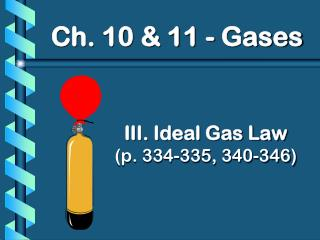Download PresentationIII. Ideal Gas Law (p. 334-335, 340-346)III. Ideal Gas Law (p. 334-335, 340-346) - PowerPoint PPT Presentation

Download PresentationIII. Ideal Gas Law (p. 334-335, 340-346)
An Image/Link below is provided (as is) to download presentation

Download Policy: Content on the Website is provided to you AS IS for your information and personal use and may not be sold / licensed / shared on other websites without getting consent from its author. While downloading, if for some reason you are not able to download a presentation, the publisher may have deleted the file from their server.

- - - - - - - - - - - - - - - - - - - - - - - - - - - E N D - - - - - - - - - - - - - - - - - - - - - - - - - - -
Presentation Transcript

1. Ch. 10 & 11 - Gases III. Ideal Gas Law(p. 334-335, 340-346)

2. Quantities to Describe Gases • P: Pressure • V: Volume • T: Temperature (Kelvin!) • n: # of moles

3. Avogadro’s Principle V n • Equal volumes of gases contain equal numbers of moles • at constant temp & pressure • true for any gas

4. Ideal Gas Law = k V n Merge the Combined Gas Law with Avogadro’s Principle: PV T PV nT = R UNIVERSAL GAS CONSTANT R=0.0821 Latm/molK R=8.315 dm3kPa/molK You don’t need to memorize these values!

5. Ideal Gas Law PV=nRT (listen to song!!!) UNIVERSAL GAS CONSTANT R=0.0821 Latm/molK R=8.315 dm3kPa/molK You don’t need to memorize these values!

6. Ideal Gas Constant, R • We know that: • 1 mol of a gas occupies 22.4 L at STP (273.15 Kand 101.325 kPa) • R = PV = (101.325kPa)(22.4L) Tn (273.15K)(1mol) R = 8.31 L·kPa/mol·K

7. Ideal Gas Constant, R • Units of numerator depend on: • Unit of volume and pressure • Common units of R 

8. Ideal Gas Law Problems • Calculate the pressure in atmospheres of 0.412 mol of He at 16°C & occupying 3.25 L. GIVEN: P = ? atm n = 0.412 mol T = 16°C = 289 K V = 3.25 L R = 0.0821Latm/molK WORK: PV = nRT P(3.25)=(0.412)(0.0821)(289) L mol Latm/molK K P = 3.01 atm

9. Ideal Gas Law Problems WORK: 85 g 1 mol = 2.7 mol 32.00 g • Find the volume of 85 g of O2 at 25°C and 104.5 kPa. GIVEN: V=? n=85 g T=25°C = 298 K P=104.5 kPa R=8.315dm3kPa/molK = 2.7 mol PV = nRT (104.5)V=(2.7) (8.315) (298) kPa mol dm3kPa/molKK V = 64 dm3

10. M from IGL • a) If the P, V, T, and mass are known for a gas sample, then n can be calculated using IGL • Then, the molar mass is found by dividing the mass by the number of moles

11. M from IGL • b) The number of moles (n) is equal to mass (m) divided by molar mass (M) g ÷ g = g x mol = mol mol g c) Substitute m/M for n in IGL: PV = mRT OR M = mRT M PV

12. D from IGL • Density, D, is m/V which results in: M = DRT P Rearranging for D: D = MP RT